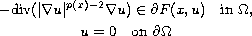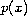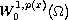Electron. J. Diff. Equ., Vol. 2010(2010), No. 44, pp. 1-9.

### Existence and multiplicity of solutions for a differential inclusion problem involving the p(x)-Laplacian Guowei Dai

Abstract:which involves the-Laplacian. By applying the nonsmooth Mountain Pass Theorem, we obtain at least one nontrivial solution; and by applying the symmetric Mountain Pass Theorem, we obtain k-pairs of nontrivial solutions in.Guowei Dai Department of Mathematics, Northwest Normal University Lanzhou, 730070, China email: daigw06@lzu.cn